Ponomarev, D. A., Mettee, H. D., and Miller, J. (2015). "Empirically estimated heats of combustion of oxygenated hydrocarbon bio-type oils," BioRes. 10(2), 3638-3656.

#### Abstract

An empirical method is proposed by which the heats of combustion of oxygenated hydrocarbon oils, typically found from wood pyrolysis, may be calculated additively from empirically predicted heats of combustion of individual compounds. The predicted values are in turn based on four types of energetically inequivalent carbon and four types of energetically inequivalent hydrogen atomic energy values. A method is also given to estimate the condensation heats of oil mixtures based on the presence of four types of intermolecular forces. Agreement between predicted and experimental values of combustion heats for a typical mixture of known compounds was ± 2% and < 1% for a freshly prepared mixture of known compounds.

#### Full Article

Empirically Estimated Heats of Combustion of Oxygenated Hydrocarbon Bio-type Oils

Dmitry A. Ponomarev,a Howard D. Mettee,b,* and J. Miller c

An empirical method is proposed by which the heats of combustion of oxygenated hydrocarbon oils, typically found from wood pyrolysis, may be calculated additively from empirically predicted heats of combustion of individual compounds. The predicted values are in turn based on four types of energetically inequivalent carbon and four types of energetically inequivalent hydrogen atomic energy values. A method is also given to estimate the condensation heats of oil mixtures based on the presence of four types of intermolecular forces. Agreement between predicted and experimental values of combustion heats for a typical mixture of known compounds was ± 2% and < 1% for a freshly prepared mixture of known compounds.

Keywords: Heating values; Heats of combustion; Empirical equation; Condensation correction; Oxygenated hydrocarbons

Contact Information: a: St. Petersburg Forest Technical University, St. Petersburg, 049128, RF; b: Chemistry Department, Youngstown State University, Youngstown, Ohio 44555 USA; c: Chemical Engineering Program, Youngstown State University, Youngstown, Ohio 44555 USA;

* Corresponding Author: hdmettee@ysu.edu

INTRODUCTION

Shifting the world’s fuel platform from non-renewable, carbonaceous fuels to renewable, hydrogen based alternatives may be desirable, but it is clear that some form of carbon-containing fuels will be a part of the energy supply chain for the foreseeable future. One source of renewable liquid fuels is mixtures of partially oxygenated bio-oils resulting for example from the pyrolysis of wood or other biomass materials. Their energy content may be, gram for gram, less than that of their pure fossil based counterparts, but bio-oils are produced from the constantly renewing process of photosynthesis, a natural carbon dioxide sink. If such oxygenated hydrocarbon oils are to be a part of tomorrow’s energy stream as a bridge fuel for the future, it would be useful to be able to characterize the energy content of such fuel mixtures rapidly and conveniently, without having to use a bomb calorimetric measurement for each mixture, and without sophisticated computations that are not always available to producers.

Historically Benson’s methods (1958, 1965, 1976) using the enthalpic values of various C-H, C-C, C-O (etc.bond energies were used to estimate the energy production from given fuel molecules, especially where the end products can be identified as CO2(v) and H2O(l), those of complete combustion. However bond energies are usually averaged over a variety of molecules, and thus their uncertainties can be more than just a few percent. Liebman (1986) later proposed using macroscopic energy values of group additivities for common molecular clusters of atoms.

An alternative approach is to assign certain empirical energy factors to each element of C, H, O, and S atoms in an often complex molecular mixture. The final states following complete fuel combustion are CO2(g), SO2(aq), and H2O(l), as before and the initial states are simply the original atoms in their parent molecules, independent of their particular molecular configuration. Here the weight per cent composition analysis is done on the mixture. This is tantamount to assuming that the atoms of each element are energetically equivalent. In the nineteenth century Pierre Dulong (1785-1838, in Capareda 2011) and Dmitri Mendeleev (1897) proposed such empirical heating value equations, where is the heat of combustion, often rendered as ΔfHo or ΔHfo (kJ/kg),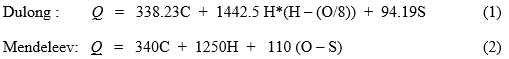and C, H, O, and S are weight percentages of those elements in the dry sample.

Subsequent to these early suggestions a number of other such correlations have appeared, some restricted to coals, or to solids, others to liquids and gases, and still others to particular biomass extracts from certain geographic regions (e.g. Spanish olive stones (Meta-Sanchez 2013)). Channiwala and Parikh (2002) critically reviewed 22 ultimate empirical correlations using 275 experimental data points, over the entire spectrum of fuel types of solids, liquids and gases, biomass and char, leading to equation (3) as the most suitable “unified correlation” to predict the heating value for this representative fuel set (kJ/kg), with an average absolute error of 1.45%,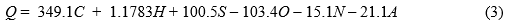where A is the residual ash content

Later the Channiwala group (2005) pointed out that a proximate analysis might be more useful in those cases where more demanding, precise elemental analysis could not be conveniently performed. Using 100 solid fuels, this correlation’s parameters (4) include the more easily calculated fixed carbon (CF), determined from the measured volatile matter (MV), and ash (A) on a dry weight basis (kJ/kg), with an average error of 3.74% . (The fixed carbon is calculated from the initial dry weight minus those of the measured moisture, volatile matter and ash fractions, using straightforward ASTM methods.)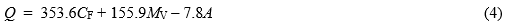Eight years ago (Parikh et al. 2007) it was pointed out that the more simply obtained proximateanalysis results could be mathematically transformed into the elemental parameters of the ultimate analytical scheme by a set of linear equations for C, H, and O for biomass fuels such that, in MJ/kg, CFMV in wt. %, one obtains: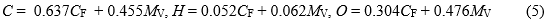The associated average absolute error for the respective elements are 3.21, 4.79, and 3.4%, which would propagate into Eq. 4, increasing its error from 1.45%, but it is still more convenient to use less expensive equipment and less trained technicians to perform this proximate analysis.

Recently Garcia et al. (2014) and Pizarro (2014) applied the ultimate and proximate techniques to 100 Spanish biomass samples including fuels, forest, agricultural, and industrial wastes, energetic crops, and cereals. Their predictive equations resulted in average absolute errors in the range of 5 to 6% in predicting HHVs (higher heating values, where the combustion water is H2O(l)) compared to their calorimetrically measured values. There is little doubt the large range of sample origins contributed to their expanded error limits.

The above mentioned methodologies depend fundamentally on either a precise knowledge of the elemental composition of a given mixture, or the numbers and types of bonds present usually in a given molecule, let alone the question of a mixture of perhaps hundreds of compounds.

The unusual approach attempted in the present work considers the energy states of types of carbon and hydrogen atoms in various configurations of hybridization and bonding arrangements in a given pure compound. Then, to estimate the HHV (abbreviated in this work as Q) for the oxygenated hydrocarbon, we rely on the weight fractions of these particular energized atoms. For a complex mixture of such compounds, as analyzed routinely using GC/MS fitted with a library which reliably identifies the compound from each GC peak, one obtains the molecular weight (and structure) directly. Such instruments, while they are not inexpensive, are easily operated. A computer spread sheet such as Excel® can then simply list each compound, and its experimental Q if known, or its computed Q based on its structure (types of energized C and H atoms) if not reliably known, and the HHV for the mixture (kJ/kg) is calculated by mass weighting each compound’s HHV, converting each from the molefraction given by the MS intensities.

The analysis outlined below shows that the energies of four types of carbon and four types of hydrogen atoms are sufficient to predict the known heats of combustion of a wide variety of oxygenated hydrocarbons to within a few percent, including small corrections for heats of condensation. Equation (5) gives the predicted combustion heat of a compound (kJ/kg) from the weight fractions of the atomic types given in square brackets. The hybridization state of the carbon atoms, spn, and its bonding partners represent changes in these compounds and these would be expected to change its energy content.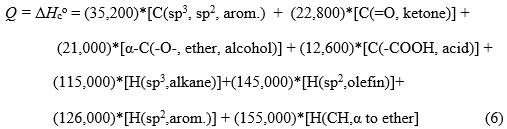The following sections show the stepwise process of determining the specific energy values for different types of carbon and hydrogen atoms found in saturated and aromatic hydrocarbons, ethers, ketones, alcohols, carboxylic acids, and esters. Since these values are determined for gas phase molecules, and pyrolitic oils are liquids, we also determine a correction for condensation to account for the slight loss in heating value when the liquids are combusted. This difference is often smaller than the precision uncertainty with which calorimetric measurements are reported, and thus might be of limited practical significance. It is still an important conceptual difference that is applicable to more precisely known cases.

EXPERIMENTAL

Heats of Combustion

Heats of combustion were measured with a traditional Parr 1672 Oxygen Bomb Calorimeter fitted with the Precision Thermometer (S/N 175) measuring digitally to the nearest 0.0001 oC. Two mixtures were prepared by weight using a Mettler Toledo EL204 balance (d = 0.0001g). The first consisted of 12 representative oxygenated organic compounds like those found in common biofuels, taken from previously opened bottles in laboratory use. Because of the possibility of moisture contamination, a second mixture was made up consisting of 7 representative compounds taken from Sigma-Aldrich Anhydrous/ ACS Reagent/ Reagent Plus Grades, opened just prior to the experiment. (The levels of purity were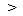99% for all except acetic anhydride (98%). The technique is familiar to any physical chemist and can be found in various current editions of Experiments in Physical Chemistry(Garland et al. 2009). The measured heat capacity of the calorimeter using the benzoic acid standard was 10.20 ± 0.05 (± 0.5%) kJ/oC.

Least Squares Coefficients

In general the procedure used began with simple hydrocarbons and then the addition of functional groups, in each case taking as many reliably known heats of combustion, usually in the gas phase, but if not correcting to it with a liquid phase value and a heat of vaporization. These input data were the reliably known (NIST WebBook, 2014) heats of combustion, QH, for these compounds, and the fractional composition of the molecular weight due to atoms of a certain type. For example, the methane molecule has a C(sp3) fractional mass composition of 0.750 and 0.250 for H(sp3). The methane equation looks like 55,669 kJ/kg = (0.750)*X + (.250)*Y, and this with eight other similar equations are solved simultaneously, in a linear least squares vector routine by PSI© (PolySoftware International, 2010) to determine the best fitting X and Y energy contents for this group of C and H atoms in the set (along with their ± standard deviations as a measure of the quality of the fit). The R2value for this line is very close to 0.9999, indicating an acceptable correlation for the model. Lastly the X and Y energy contents were used to calculate individual Qvalues for each compound. individual Qvalues for both C and H atom types found in alkanes, alkenes, ethers, aromatics, alcohols, ketones, phenols, etc., sometimes using X and Y values previously determined from simpler molecules.

RESULTS

Alkane Prototype

The results for alkanes are listed in Table 1 and Fig. 1, which demonstrate the correlation between the experimental and calculated values using the best fit energy values X and Y for C (sp3) atoms and their bonded H atoms, respectively, for the nine simultaneous linear equations.

Table 1. Known Heats of Combustion (QH) (NIST 2014) for Alkanes, Fractional C and H Weights, and Calculated QH Using Equations QH (or QHCalc) = FrC*X + FrH*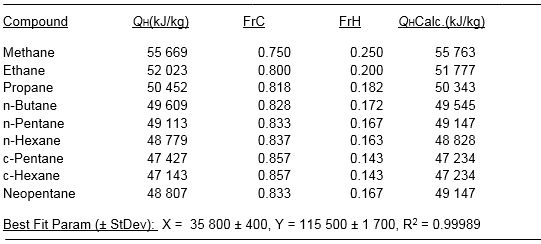It can be pointed out that the calculated heat of combustions based on the best fit X and Y parameters agreed to within ± 0.27 % of the experimental values, well within the precision limits of most acceptable calorimetrically determined quantities.

Alkenes With sp2 and sp3 Carbons

There are few common olefins with only sp2 carbons and hydrogens, and whose heats of combustion have been measured carefully – namely ethylene, 1,3-butadiene and 1,3,5–hexatriene. Nevertheless three simultaneous linear equations of the type QH = FrC*X’ + FrH*Y’ may be solved as before to determine the possibly new sensitivity factors X’ and Y’ for sp2 C and H atoms attached thereto.

Table 2 summarizes the results, where it is observed that the energy content per kg of C atoms is nearly the same as for alkanes, but that for the sp2 H atoms is noticeably greater. The cooperative stabilizing effect of electron delocalization does not appear to be significant here from the carbon standpoint, but the H atoms appear to be more destabilized relative to those in alkanes.

Table 2. Heats of Combustion (QH) for Olefins (NIST 2014) Fractional C and H Weights, and Calculated QH Using Equations QH (or QHCalc) = FrC*X’ + FrH*Y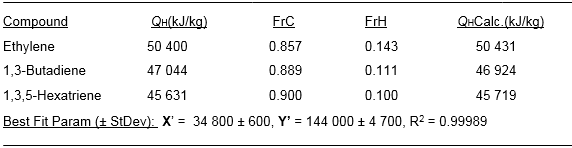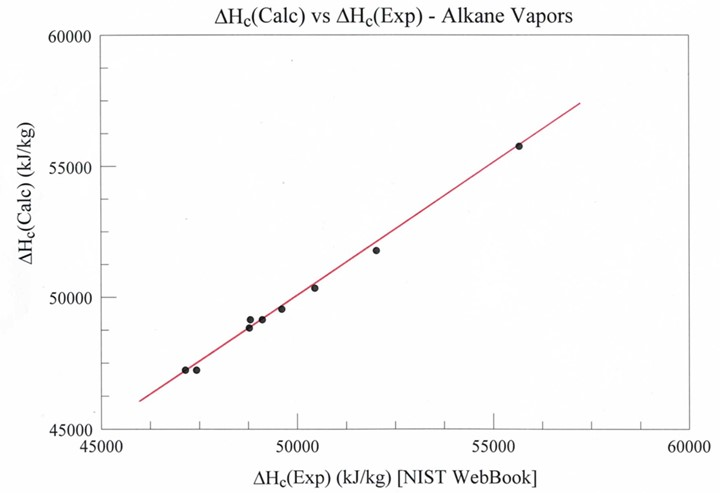Fig. 1. Experimental heats of combustion of nine simple alkane vapors (C1-C7) used to simultaneously solve nine equations of the form ΔHc(Exp) = 0.0 + FrC*(X) + FrH*(Y), where X and Y are the least square energy coefficients for sp3 carbon and their bonded hydrogen atoms, respectively. ΔHc(Calc) is calculated using X (35,800 kJ/kg) and Y (115,500 kJ/kg) as the best fit energy coefficients for C and H atoms.

Alternatively, when both sp2 and sp3 carbons occur in the same molecule, there are four types of atoms to be considered, so that at least four compounds need to be included to find the coefficients X, X’, Yand Y’. If propylene, 1-butene and E and Z 2-butene are added to the three “pure “ (sp2) olefins, one can solve independently for another set of these coefficients, as is done and presented in Table 3.

The results of Table 3 support the energy values for X’ and Y’ (sp2 C and H’s) given in Table 2 using only sp2 carbons. The larger deviations for X and Y values for the sp3 C and H’s sensitivities do not suggest changing them from those already obtained using the nine pure alkanes (Table 1).

Aromatic Compounds

The seven aromatic compounds chosen as reference compounds to calibrate the energy content of aromatic carbons and their associated hydrogen atoms sometimes have alkyl groups attached. Instead of including their contributions as unknowns, the alkyl contribution was subtracted out from the measured heat of combustion using earlier found sensitivities. Then these “adjusted” heats of combustion were used in an equation containing only aromatic C and H fractional weights. The results are summarized in Table 4.

Table 3. Heats of Combustion (QH) for Mixed Alkane-Olefin Vapors (NIST 2014), Fractional C and H Weights, and Calculated QH Using Equations Q(or QHCalc) = FrC*X + FrH*Y + FrC’*X’ + FrH’*Y’. (‘ refer to sp2 C and H)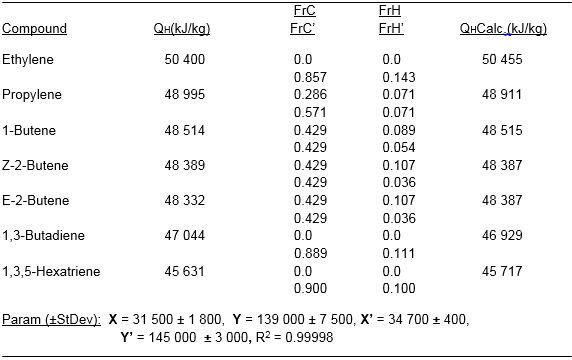Table 4. Heats of Combustion (QH) (NIST 2014) and Adjusted QH (see text), Fractional C and H Weights, and Calculated QH Using Equations Q(or QHCalc) = FrC*X” + FrH*Y”.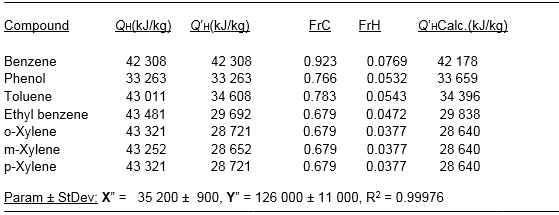The first conclusion from Table 4 is that the aromatic C atom energy content was within experimental error of those of the alkane and olefinic carbon atoms (35 800 and 34 800 kJ/kg, respectively). Secondly, despite the 9% precision error associated with aromatic H atom’s energy content, it is different than those H atoms associated with spC-H’s (115 500) and sp2 C-H’s (145 000 kJ/kg).

Added Alkyl Ether, Alcohol, and Ketone Functional Groups

Perhaps the simplest extension to the molecular structures considered so far is to insert either an ether O atom or a carbonyl group (ketone) in a carbon chain or attached to a ring to see if the adjacent (α) carbon or hydrogen atoms are affected as far as their energy state is relative to CO2 or H2O, respectively. For example dimethyl ether, methanol or acetone’s heat of combustion would manifest the O atom’s effect on the included C (ketone) or adjacent C’s and H’s, α, in alcohols or ethers, while those atoms more remote from the O atom could be considered as in the previous hydrocarbons.

Possibly the case of alkyl ethers is the simplest next step, since only α-Cs, (Cα) atoms adjacent to the O atom, and their attached α-Hs, (Hα) atoms are present, along with the more remote Cr and Hr atoms. Here there are two nearly equivalent approaches. The first is to consider the fractional weights of the two types of both carbon and hydrogen atoms and solve for the four adjustable energy contents. (No energy is assigned to the O atom itself.) Table 5a contains the results for five aliphatic ethers using this method. The second (and not independent) method is to adjust the experimental heat of combustion (as for the alkylated aromatics) by subtracting out the contributions due to the remote Cr and Hr atoms using the earlier determined values (Table 1), and then solve the set of equations for the Cα and Hαcontributions. Table 5b shows these results.

Table 5a. Heats of Combustion (QH) (NIST 2014) for Five Aliphatic Ethers , Fractional Cα and HαWeights, and the Remote Cr and Hr Fractional weights, in the Equations QH(or QH(calc) = FrCα*X’ + FrHα*Y’ + FrCr*X + FrHr*Y.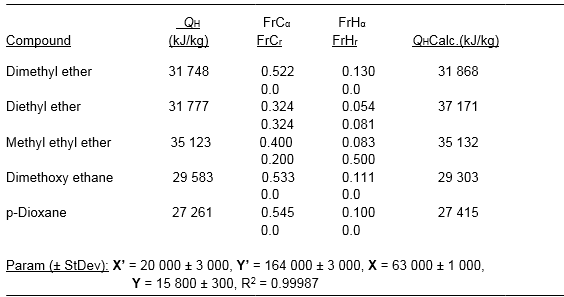Table 5b compares the data for these five aliphatic ethers, first recalculating
the experimental combustion data (NIST 2014) to remove the contributions from the remote (non α) C and H atoms, leaving five equations and two unknowns, the energetic sensitivities due to the Cα and Hα atoms (X’ and Y’).

Table 5b. Adjusted Heats of Combustion (QH) [see text] for Five Alkyl Ethers and Fractional Weights for Cα and Hα Atoms in the Equations QH (or QHCalc) = FrCα*X’ + FrHα*Y’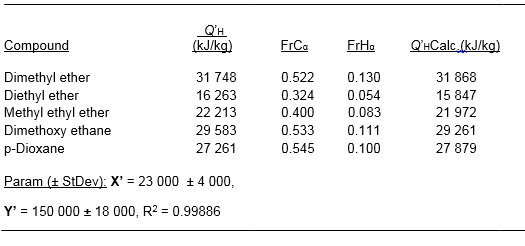The results of Tables 5a and 5b show a growing percentage uncertainty in the sensitivity coefficients for C and H atom adjacent to sp3 O atoms, but sufficiently outside the error range for the ordinary (remote) aliphatic C (35 800 ± 400 kJ/kg) and H (115 000 ± 1 700). It was possible to assign different average values of Cα (21 000 ± 3 000) and Hα(155 000 ± 15 000) for these alkyl ethers using both sets of data. The predicted heats of combustion were still within a few percent of the actual (or adjusted) values.

Table 6 lists the heats of combustion of six aliphatic ketones, where the distinctive sp2 carbonyl carbon may be singled out as an unknown and the aliphatic C and H energies can be separately calculated. A value of zero is assigned for the already “oxidized” spO atom, and the resulting carbonyl carbon energy is then determined by difference. Then the average sensitivity per C(sp2) atom is found by averaging the energy differences per kg of each compound, after each is divided by the weight fraction of that kg due to C(sp2). Thus each kg of ketone C(sp2) atom releases 22 800 ± 600 kJ/kg, (a 2.6 % precision error). This value is distinctly different than the one found for olefinic C atoms (34 800).

The combustion energy values for common alcohols are given in Table 7, where the distinction between α-C and α-H atoms (w.r.t. the oxygen) versus the remote Cr and Hr atoms as for the ethers, is not only not necessary but also counterproductive. If the combustion heat is divided among four adjustable parameters, poor correlations result. As a test one might try to predict the combustion heats of the next two members in the series, 1-pentanol and 1-hexanol using only the least square sp3 C and H energy parameters in Table 7, which are not remarkably different than those given in Table 1 for simple alkanes. The corresponding predicted heats of combustion are 38, 143 kJ/kg for 1-pentanol and 39, 216 kJ/kg for 1-hexanol.

The respective experimental values (corrected for vaporization) are 38,499 and 39,667 kJ/kg, differing by 0.9% and 1.1% from the calculated ones. It is also not necessary to include the alcoholic H atom.

The close correspondence of these parameters to those of normal alkanes suggests that for alcohols there is not the same distinction between αC and αH atoms as was found for ethers.

Table 6. Heats of Combustion (QH) for Aliphatic Ketone Vapors (NIST 2014), Fractional C and H Weights, Excluding the C (sp2) Contribution, Found by Difference (see text)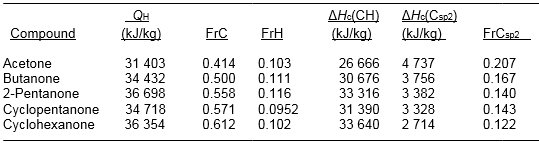Table 7. Heats of Combustion (QH) (NIST 2014) for Five Alcohol Vapors, Fractional Weights for the C and H Atoms (excluding OH) in the Equations (or QHCalc) = FrC*X + FrH*Y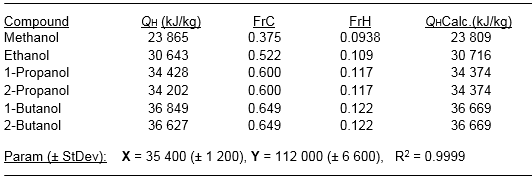Carboxyl Group

The procedure here involves considering the combustion heats of six homologous acid vapors from formic to hexanoic in the same manner as that for the ketones. This involves taking the experimental heats of combustion known mostly for the liquids, adjusting them with the heats of vaporization where needed (again using NIST tabulated data; NIST 2014), subtracting the heats of combustion contributed by the non-carboxyl fragment, and averaging the normalized remainders due to the sp2 C in the carboxyl group, now almost completely oxidized. Table 8 summarizes the relevant information.

Table 8. Heats of Combustion (QH) for Aliphatic Acid (NIST, 2014) Fractional C and H Weights (and C(sp2)), and Hydrocarbon ΔHc(CH). ΔHc(Csp2) Found by Difference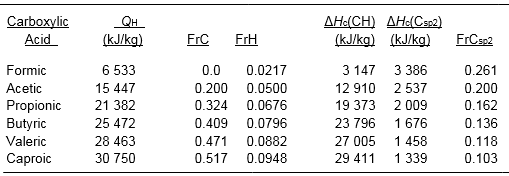For formic acid (MW 46 amu) only, the C(sp2) H atom kJ contribution is (1/46)*(145,000 kJ/kg), the olefinic value. For the other compounds, the C(sp3) and associated H’s have their usual energy values of 35,800 and 115,000 kJ/kg, respectively.

The average sensitivity per C (sp2) atom is found by taking the energy differences per kg for each compound, each divided by the fraction of the kg due to C (sp2) before averaging. Thus each kg of acidic -(C=O)-O carbon atoms release 12 600 ± 200 kJ/kg (a 1.6 % precision error, based on mean deviations). This value would presumably apply to esters as well.

Intermolecular Effects – Dispersion and Dipolar Forces

Oxygenated pyrolitic liquids most commonly contain alcohols, phenols, ethers, acids, and even pure hydrocarbons in liquid solution. To estimate their combustion heats from a known composition, no matter how many components, one needs to convert a calculated vapor phase combustion heat (on which the present numerical values are based) to a smaller value because of the stabilization energy each compound experiences due to intermolecular attractions in the liquid mixture. Such a “condensation corrected” value for the mixture’s heat of combustion would correspond to that measured in an oxygen bomb experiment with the particular oil.

The solvent stabilization energy would strictly be uniquely dependent on the oil composition, but the condensation energy released for each pure compound going from vapor to liquid (inverse of the heat of vaporization) can give a reasonable idea of the magnitude of this small correction. It is recognized that these intermolecular forces result from London (or dispersion or exchange), dipole-dipole and hydrogen bonds, sometimes collectively known as van der Waals forces, but all three contribute to the heat of vaporization. We chose to consider these types separately by looking at groups of representative molecules which contained only one, or two, or all three forces.

Table 9a collects the heats of vaporization of alkyl and aromatic hydrocarbons from C3 to C8, as taken from the most recent NIST WebBook (2014) values, to assess the dispersion force effect in the absence of polar and H-bonded groups of molecules in the size range of components of wood pyrolitic oil substituents.

Table 9a. Heats of Vaporization (NIST 2014) of Simple Mixed Hydrocarbons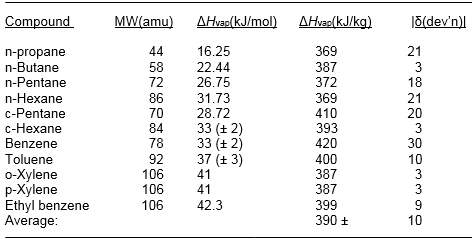It is interesting to see that this dispersion component of the heat of vaporization is relatively constant on a mass basis while generally increasing on a molar basis.

Table 9b collects the heat of vaporization information for some simple C3 – C6 ketones (K) and butanal. These values are compared to the corresponding hydrocarbon to separate out the carbonyl dipole-dipole intermolecular effects from the dispersion based attractions. This subtracts out the hydrocarbon contribution [CH] from the total heat of vaporization, leaving the dipole-dipole from the carbonyl groups as a remainder.

Despite the usual understanding that dipole-dipole attractions are stronger than non-polar dispersion forces, it appears in this group of molecules that the polar carbonyl groups contribute approximately 20% of the effect of these dispersion forces. Finally, we may assume this same dipolar-dipole effect to apply to carboxylic acid and ester groups.

Table 9b. ΔHvap for Representative Carbonyls (NIST 2014) Compared to the Corresponding Hydrocarbon [CH] (Table 9a), and the Carbonyl Difference [C=O]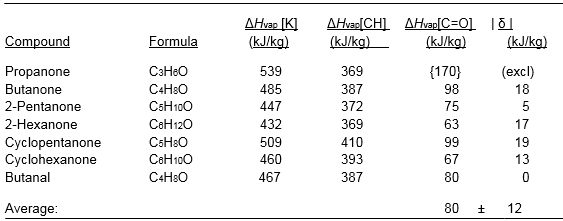Intermolecular Effects – Hydrogen Bonds

Tables 10a and 10b list the heats of vaporization of several alcohols [A] and phenols [P] to assess the effect of hydrogen bonding intermolecular forces in the process of vaporizing these molecules. Again the hydrocarbon exchange contribution to the intermolecular attraction is factored out as before, leaving the H-bond isolated.

Table 10a. ΔHvap for Representative Alcohols [A] (NIST 2014) Compared to the Corresponding Hydrocarbon, CH, (Table 9a), and the H-Bond Difference (H∙∙∙O)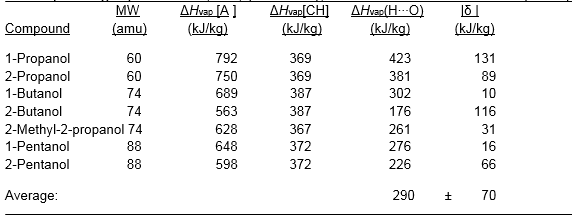Table 10b.
ΔHvap for Representative Phenols [P] (NIST 2014) Compared to the Corresponding Hydrocarbon, CH, (Table 9a) and the H-Bond Difference (H∙∙∙O)
(a Lange’s Handbook 1985)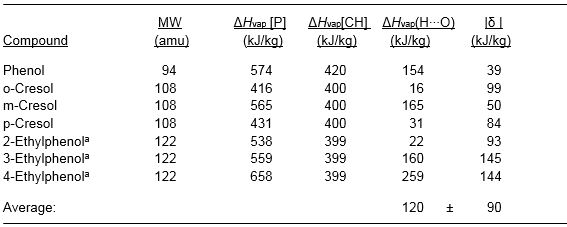Comparing Tables 10a and 10b, it appears the alcoholic groups add more to the heat of vaporization of the corresponding parent hydrocarbon than the phenolic OH adds to the heat of vaporization of the corresponding parent aromatic ring. The overall conclusion one may draw from this section is that even when all three intermolecular forces are engaged in reducing a heat of combustion from its value in the gas phase to that in liquid phases, their combined effect is not more than 900 ± 200 kJ/kg, for these types of molecules. Experimental precision limits of many calorimetric values of combustion heats in the literature are not infrequently larger. However, to be correct when comparing heats of combustion predicted by the present empirical theory (based on gas phase molecules), to those experimentally measured for liquid phases, a small correction should to be made to reduce the heat of combustion for the liquid due to condensation.

Summary of Results

Tables 11a-c summarize the sensitivity factors for the types of C and H atoms, and the condensation correction, which may be used to estimate the molecular heats of combustion for the oxygenated hydrocarbon compounds found in typical bio-type oils.

Table 11a. Summary Energy Values Found for C Atom Types in Oxygenated and Pure Hydrocarbons. (n) reference points used for each determination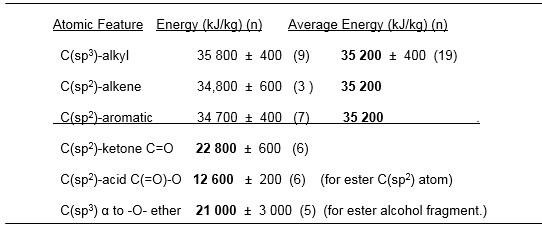Table 11b. Summary Energy Values for H Atom Components of Oxygenated
and Pure Hydrocarbons. (n) defined in 11a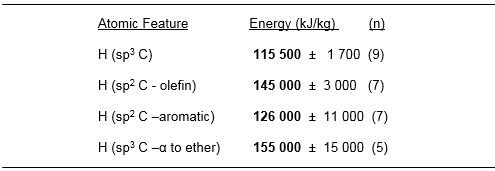Table 11c. Intermolecular Condensation Energies for Hydrocarbons and Oxygenated Hydrocarbons. (n) defined in 11a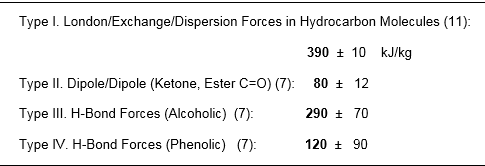The three slightly different values for sp3 carbon atoms in hydrocarbons (remote from O atoms) may be averaged for convenience without significantly altering the net outcome. For precise estimates the condensation correction should be made as noted above.

Application and Experimental Verification

Figure 2 compares the experimental (NIST 2014) and empirically predicted values of the heats of combustion of 38 compounds of the type typically found in pyrolitic oils. The predicted ΔHc came within 2.5% of the actual values over all 38 and within 1.5% after 6 outliers (incl. glycerol, ascorbic and lactic acids) were excluded. The question of whether the method succeeds for arbitrary mixtures requires experiments. The following section compares the calorimetrically measured heats of combustion of two liquid mixtures with those calculated additively from the empirical model.

For reference, the heats of combustion of these mixtures are also calculated from the additive heats of combustion of the individual components taken from the NIST calorimetric tables. The question might arise, “why bother,” since the calorimetric values are already available. The answer is that of the many oxygenated hydrocarbon compounds found in bio-type oils, a majority of their heats of combustion have not yet been reliably measured.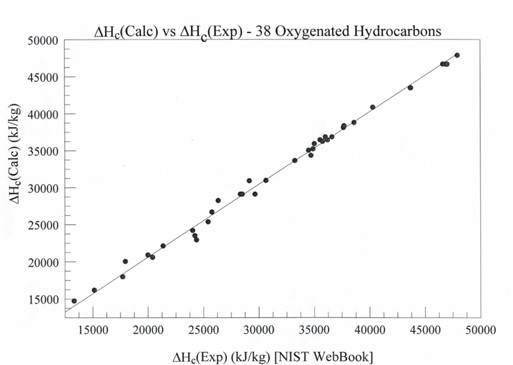Fig. 2. ΔHc(Calc) vs. ΔHc(Exp) (NIST, 2014) for 38 common oxygenated hydrocarbons resembling those found in pyrolitic oils. The equation is ΔHc(Calc) =0.0 + FrC1*(a1) + FrC2*(a2) + FrC3*(a3) + FrC4*(a4) + FrH1(b1) + FrH2(b2) + FrH3(b3) + FrH4*(b4). The FrCi and FrHi are the fractional molecular weights of carbon and hydrogen atoms of types 1-4 (see text). The coefficients a1 and bi are the atomic energy values (kJ/kg) for these types of atoms.

Table 12 presents the relevant calorimetric data, where the (±) uncertainties are simple averaged mean deviations over 3 to 4 trials, also given as a (%) precision. Looking at the results for Mixture 1, the “off the shelf” compounds in use for an uncertain number of months, both the predicted additive value from the NIST WebPage (NIST 2014) and that given by the empirical theory presented in this paper, are 5.8% and 3.8 % greater, respectively, than the experimentally measured heat of combustion. The approximate agreement between the two predicted values suggests the possibility that a small amount of moisture may have entered these reagent bottles, thereby reducing its calorific value. Therefore Mixture 2 was prepared using freshly opened minutes before the experiment and giving more precise results closer to predicted ones.

Table 12. Thermal Data for Test Mixtures vs. Predicted Combustion Heats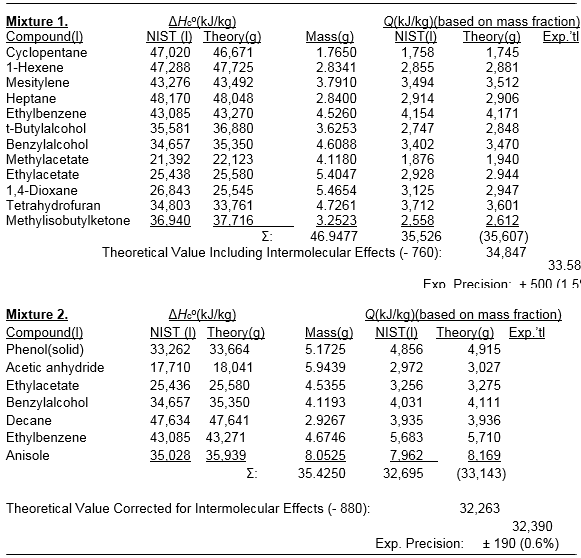Table 12 shows the results for Mixture 2 including the added calorific values for each compound (liquid) to give a heat of combustion 0.9% greater than that actually measured, while the empirical theory gave the same quantity 0.4% less than the measured value. (The precision of the measured value is greater than for Mixture 1 probably because the procedure of calculating the small weight loss due to evaporation during sample preparation between weighing the mixture and its closure in the oxygen bomb (typically 45 sec) was better controlled for the second mixture.)

Both calculated values were within 1 % of the actual value, perhaps better than some of the measured calorimetric values upon which the sensitivity factors were based, or some of the experimental values used to compare to the empirically predicted ones by the method suggested here. If the accuracy for these known mixtures of compounds carries over to those compounds for which heats of combustion have not yet been reported, then there is a method to estimate it for any pyrolitic oil mixture to within 1 to 2%, for which reliable GC/MS analysis is available.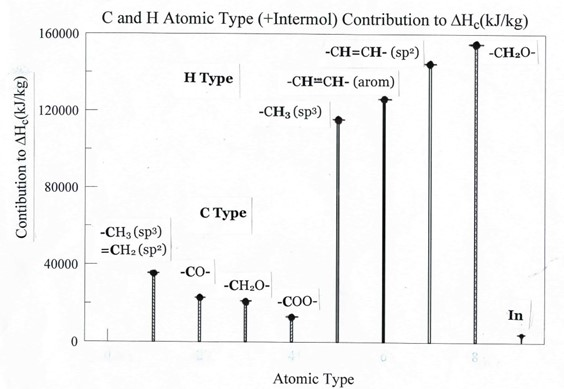Fig. 3. Histogram of carbon atom and hydrogen atom energy values (kJ/kg) empirically derived from least square solutions of NIST WebBook standard heats of formation of familiar compounds (see text). Oxygenation causes a loss available energy from O-attached carbon atoms, while oxygenation and unsaturation enhances the available energy from hydrogen atoms attached to these carbons. The intermolecular effect (In) is nearly always two orders of magnitude smaller than the combustion heats.

Figure 3 presents a comparative view of the empirical energy values of the various types of carbon and hydrogen atoms found to contribute to the standard combustion heats of hydrocarbons and oxygenated hydrocarbons typical of those found in bio-type oils from wood. The energy content per kg of carbon atoms diminishes with degrees of oxidation of that carbon atom, while that contributed by hydrogen atoms attached to those carbon atoms, or to unsaturated carbons, increases. The small intermolecular effect reduces the calculated combustion heat for the mixture, and is based upon which type of polar functional groups are present to exert them.

CONCLUSIONS

1. The present paper identifies four (4) carbon and four (4) hydrogen energy states, with respect to CO2(g) and H2O(l) at 298 K, based on their fractional mass of the molecular structure and the known molar heats of combustion (in kJ/kg). (See text for actual values and statistical methodology.)

2. Using these atomic energy values one may predict the heats of combustion contributed by each type of C and H atom in compounds in complicated mixtures of oxygenated hydrocarbon oils, given the GC/MS compositional breakdown (usually by moles, converted to kJ/kg).

3. When this method was tested with known mixtures, the results were within ± 1% of those predicted, thus making it possible to precisely calculate the heat of combustion of any mixture of these types of oils using a straight forward Excel©-type spreadsheet. This avoids the necessity of having to perform numerous calorimetric measurements.

4. Intermolecular attractions are considered and found to reduce by less than a few % the total combustion heat, as estimated empirically from the heats of vaporization of the various representative liquids, often within the experimental precision of the measurements themselves.

5. The eight different carbon and hydrogen energy states identified empirically in these oxygenated hydrocarbons point to trends that should have theoretical rationale.

ACKNOWLEDGEMENTS

The authors would like to express their gratitude to the U.S. Civilian Research and Development Foundation (CRDF) and the Russian Federation for Basic Research (RFBR) for their financial support (Grant RUC1-2989-ST-10). The Chemistry Department of Youngstown State University and the Chemistry Faculty of St. Petersburg State Forest Technical University, also generously supported these studies.

REFERENCES CITED

Benson, S. W., and Buss, J. H. (1958). “Additivity rules for the estimation of molecular properties, thermodynamic properties,” J. Chem. Phys. 29(2), 546-572.

Benson, S. W. (1965). “Bond Energies,” J. Chem. Ed. 42(9), 502-518.

Benson, S. W. (1976). Thermochemical Kinetics, 2nd Ed., John Wiley & Sons, New York.

Capareda, S. C. (2011), “Biomass Energy Conversion,” in: Sustainable Growth and Applications in Renewable Energy Sources, M. Nayeripour (ed.), InTech (Croatia), 209-226.

Channiwala, S. A., and Parikh, P. P. (2002). “A unified correlation for estimating HHV of solid, liquid and gaseous fuels,”Fuel 81, 1051-1063.

Garcia, R., Pizzaro, C., Lavin, A.G., and Bueno, J. L., (2014). “Spanish biofuels heating value estimation. Part I: Ultimate analysis data,” Fuel 117, 1130-1138.

Garcia, R., Pizzaro, C., Lavin, A.G., and Bueno, J. L., (2014). “Spanish biofuels heating value estimation. Part II. Proximate analysis data,” Fuel 117, 1139-1147.

Garland, C., Nibler, J., and Shoemaker, D. (2009). Experiments in Physical Chemistry, 8th Ed., McGraw-Hill Higher Education, New York.

Lange’s Handbook of Chemistry, 13th ed., J.A. Dean, Ed., McGraw-Hill Book
Company (1985).

Liebman, J. (1986), “Macroincrementation reactions: A holistic estimation approach for the properties of organic compounds,” in: Molecular Structure and Energetics, Vol. 3, VCH Publishers, Florida.

Mata-Sanchez, J., Perez-Jimenez, J. A., Diaz-Villanueva, M. J., Serrano, A., Nunez-Sanchez, Lopez-Gimenez, F. J., (2013). “Statistical evaluation of quality parameters of olive stone to predict its heating value,” Fuel 113, 750-756.

Mendeleev, D. I. (1897). Fundamentals of the Industrial Factory, St. Petersburg, Russia (Russian), 90.

National Institute of Standards and Technology (2014), NIST WebBook, www.NIST.gov.

Parikh, J., Channiwala, S. A., and Ghosal, G. K. (2005),” A correlation for calculating HHV from proximate analysis of solid fuels,” Fuel 84, 487-494.

Parikh, J., Channiwala, S. A., and Ghosal, G. K. (2007),” A correlation for calculating elemental composition from proximate analysis of biomass materials,” Fuel 86,
1710-1719.

PSI-Plot User Guide (2010). 15th Ed., Poly Software International, Pearl River, NY

Article submitted: December 8, 2014; Peer review completed: April 4, 2015; Revisions accepted: April 16, 2015; Published: April 28, 2015.

DOI: 10.15376/biores.10.2.3638-3656